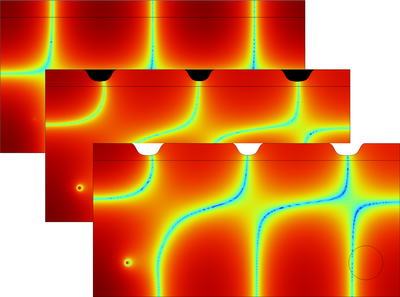# アプリケーションギャラリ

## Topology Optimization and Verification of an Acoustic Mode in a 2D Room

Application ID: 44501

This tutorial introduces the use of topology optimization in acoustics. The goal of the optimization is to find the optimal material distribution (solid or air) in a given design domain, here the ceiling of a 2D room, that minimizes the average sound pressure level in an objective region. The optimization is carried out for a single frequency. The topology optimized design is further transformed into a geometry and the results of the optimization are verified using actual sound hard boundaries.

The model requires the Optimization Module and the Acoustics Module.This model example illustrates applications of this type that would nominally be built using the following products: